# Concrete Mix Design M30 Grade with Flyash as Partial replacement to Cement : An Illustrative Example

Concrete mix design is a procedure of selecting the suitable ingredients of concrete and their relative proportions with an objective to prepare concrete of certain minimum strength, desired workability and durability as economically (value engineered) as possible.

Flyash is the most economical and easily available mineral admixture that can partially replace cement in a concrete mix ,the resultant concrete will have excellent fresh, hardened and durable properties.

As per IS:456, the maximum replacement of cement with flyash have a capping of 35%.

As we decide to go for a concrete mix design, collect the following data before hand as few design stipulation are freezed on the basis of these data.

## Preliminary Data Required for Mix Design:

Purely governed on the local conditions, were the concrete need to be applied

• Exposure Conditions of the structure: The general environment to which the concrete will be exposed during its service life, is categorized into five class to severity, as per IS 456.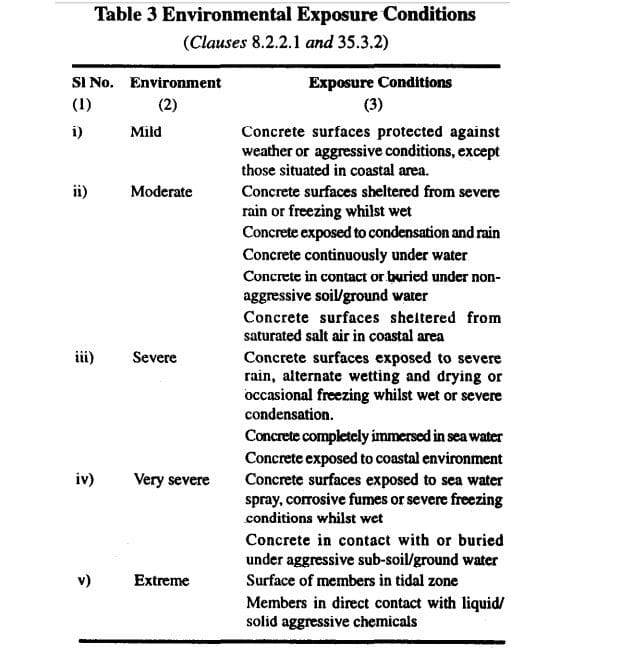The exposure condition limit the minimum cement content content (with flyash it is total cementitious content – “cement + flyash”), maximum water – cement ratio content (with flyash it is total water – cementitious content) and minimum grade of concrete.

As per exposure condition, you have the above data for working the first trial and arriving its mix proportion.

If you are getting desired result at lower cement content (with flyash it is total cementitious content), you need to put extra as freezed by IS 456.

• Minimum thickness of member: Size of aggregate should not be more than one-fourth of the minimum thickness of member, mostly 20 mm nominal size aggregate is suitable for most works. It is always suggested to go the maximum nominal size of aggregate to save on quantity of cement per unit of concrete.
• Workability: Placing condition of concrete governs its workability, low – slump of 25-75 mm (lightly reinforced sections in slab, beam, column) to high –  slump of 100-150 mm (slip form, pumped concrete) .

### Illustrated example of M30 Grade Fly Ash concrete, concrete exposed to Moderate exposure conditions.

(i) Stipulation for Proportioning Concrete Ingredients

(a) Characteristic compressive strength required in the field at 28 days grade designation — M 30

(b) Type of Cement                                                               :           OPC 53 Grade confirming to IS 12269

(c) Type of Fly Ash                                                                :           Class F

(d) Maximum Nominal size of aggregate   — 20 mm

(e) Shape of CA — Angular

(f) Workabiltiy required at site — 100 mm (slump)

(g) Type of exposure the structure will be subjected to (as defined in IS: 456) — Moderate

(h) Method of concrete placing: pump able concrete

(ii) Test data of material

The following materials are to be tested in the laboratory and results are to be ascertained for the design mix

(a) Cement Used                               :           OPC 53 Grade Confirming to IS 12269

(b) Fly Ash                                          :           Class F

(c) Specific Gravity of Cement         :           3.15

(d) Specific gravity of Fly Ash           :           2.2

(e) Chemical admixture                   :           Super plasticizer confirming to IS 9103

(f) Specific gravity

Specific gravity of Fine Aggregate (sand)   :           2.70

Specific gravity of Coarse Aggregate           :           2.80

(g) Water Absorption

Coarse Aggregate                                          :           0.4%

Fine Aggregate                                              :           1.0%

(h) Free (surface) moisture

Coarse Aggregate                                          :           Nil

Fine Aggregate                                              :           Nil

Aggregate are assumed to be in saturated surface dry condition usually while preparing design mix.

(g) Seive Analysis

Fine aggregates                                             :Confirming to Zone I of Table 4 IS – 383

## Step by Step Procedure for Concrete Mix Design of M30 Grade Fly Ash Concrete

### Step 1 — Determining the Target Strength for Mix Proportioning

F’ck = fck + 1.65 x S

Where,

F’ck = Target average compressive strength at 28 days

Fck = Characteristic compressive strength at 28 days

S = Assumed standard deviation in N/mm2 = 5 (as per table -1 of IS 10262- 2009)

= 30 + 1.65 x 5.0 = 38.25 N/mm2

Note : Under control conditions if Target average compressive strength is achieved then at field ,the probability of getting compressive strength of 30 MPa is very high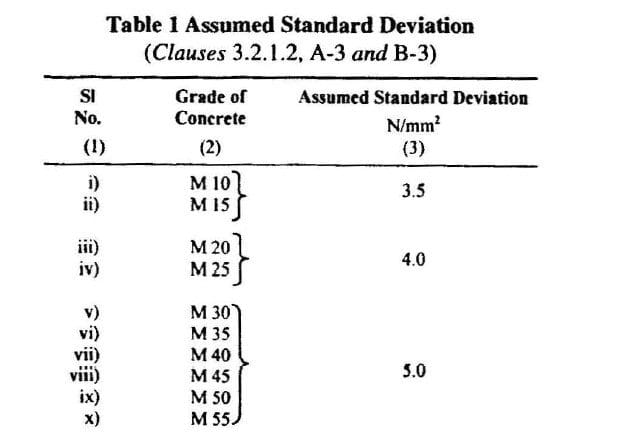### Step 2 — Selection of water-cement ratio:-

From Table 5 of IS 456, Maximum water-cementitious (w/cm)ratio = 0.50

Note : Do not start with w/cm ratio above 0.50,even though the other desired results like Strength, workability could be achieved.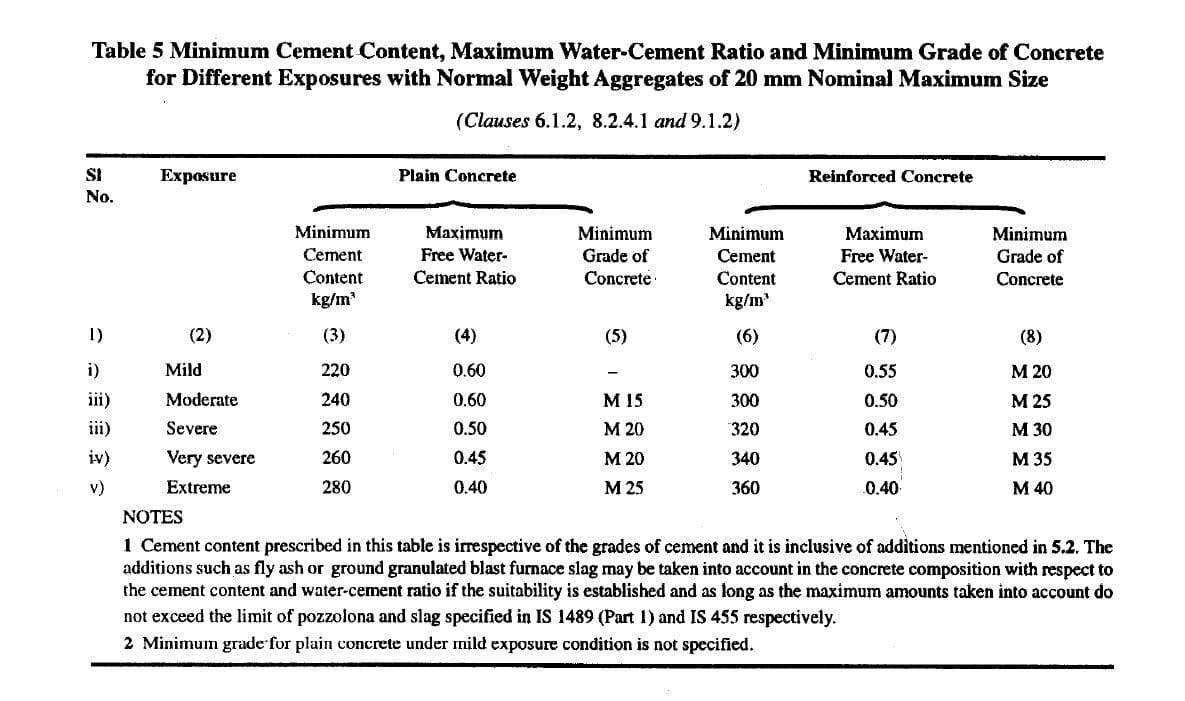### Step 3 — Selection of Water Content

From Table 2 of IS 10262- 2009,

Maximum water content for 20 mm aggregate = 186 Kg (for 25 to 50 slump)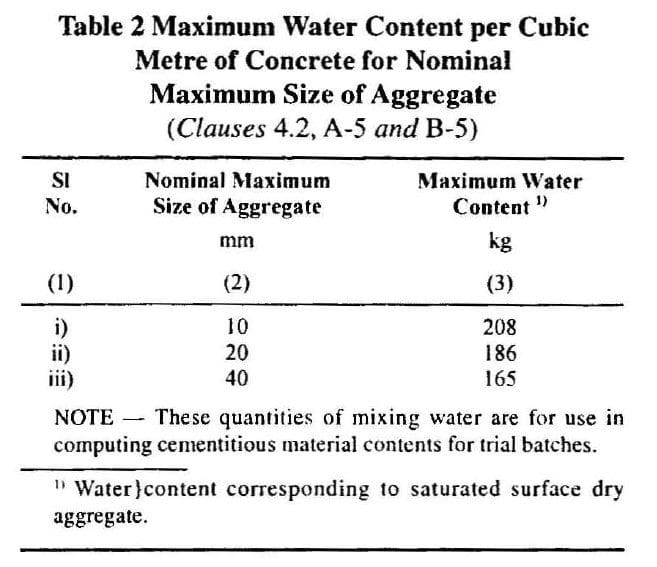We are targeting a slump of 100mm, we need to increase water content by 3% for every 25mm above 50 mm i.e. increase 6% for 100mm slump

i.e. Estimated water content for 100 Slump = 186+(6/100) X 186 = 197 litre

Water content = 197 liters

### Step 4 – Calculation of Cementitious Content

Water-Cement Ratio                         = 0.50

Water content from Step – 3 i.e. 197 liters

Cement Content = Water content / “w-cm ratio” = (197/0.50) = 394 kgs

From Table 5 of IS 456,

Minimum cement/cementitious content for moderate exposure condition = 300 kg/m3

394 kg/m3 > 300 kg/m3, hence, OK.

As per clause 8.2.4.2 of IS: 456

Maximum cement content = 450 kg/m3, hence ok too.

### Step 5: Proportion of Volume of Coarse Aggregate and Fine aggregate Content

From Table 3 of IS 10262- 2009, Volume of coarse aggregate corresponding to 20 mm size and fine aggregate (Zone I) = 0.60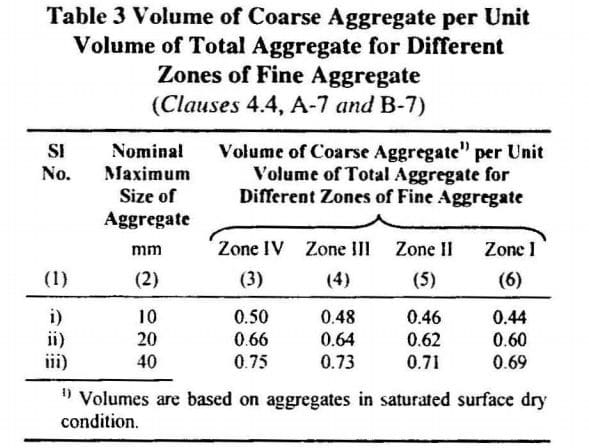Note 1: In the present case water-cement ratio is 0.5.So there will be no change in coarse aggregate volume i.e. 0.60 .

Note 2: Incase the coarse aggregate is not angular one, then  volume of coarse aggregate may be required to be increased suitably based on experience.

### Step 6: Estimation of Concrete Mix Calculations

The mix calculations per unit volume of concrete shall be as follows:

a) Volume of concrete = 1 m3

As cement is partially replaced by flyash, initially we will go for 20% replacement.

Earlier in Step 4 we got cement content of 394 kg/cum ,20% of it replaced by fly ash i.e. ~79 kg of flyash and cement content  of 315 kg/cum, keeping cementitious content of 394 as arrived in STEP 4.

b) Volume of cement = (Mass of cement / Specific gravity of cement) x (1/1000)

= (315/3.15) x (1/1000) = 0.100 m3

c) Volume of flyash = (Mass of flyash / Specific gravity of cement) x (1/1000)

= (79/2.2) x (1/1000) = 0.036 m3

d) Volume of water = (Mass of water / Specific gravity of water) x (1/1000)

= (197/1) x (1/1000) = 0.197 m3

e) Total Volume of Aggregates = 1- (b+c+d) =1- (0.1+0.036+0.197) = 0.667 m3

f) Mass of coarse aggregates = d X Volume of Coarse Aggregate X Specific Gravity of Coarse Aggregate X 1000 = 0.667 X 0.60 X 2.80 X 1000 = 1121 kgs/m3

g) Mass of fine aggregates = d X Volume of Fine Aggregate X Specific Gravity of Coarse Aggregate X 1000 = 0.667 X 0.40 X 2.70 X 1000 = 720 kgs/m3

### Step-7: Concrete Mix proportions for Trial Number 1

Cement = 315 kg/m3

Fly Ash = 79 kg/m3

Water = 197 kg/m3

Fine aggregates = 720 kg/m3

Coarse aggregate = 1121 kg/m3

Water-cementitious ratio = 0.50

Note-1: Aggregates should be used in saturated surface dry condition. If otherwise, when computing the requirement of water, allowance shall be made for the free (surface) moisture contributed by coarse aggregate and fine aggregate. On the other hand, if the aggregates are already dry, the amount of water mixed should be increased by an amount equal to the moisture likely to be absorbed by the aggregates. Necessary adjustments are also required to be made in the mass of the aggregates. The surface water and percentage water absorption shall be determined according to IS 2386.

Correction in water content due to absorption or moist aggregates

The slump shall be measured and the water content and shall be adjusted for achieving the required slump based on trial, if required. The mix proportions shall be reworked for the actual water content and checked for durability requirements.

Two more trials having variation of +/- 10% of water cement ratio  shall be carried out and a graph between three water-cement ratios and their corresponding strength shall be plotted to work out the mix proportions for the given target strength for field trials. However, durability requirement shall be met.

Two more trials having variation in fly ash replacement of 15 & 25% shall be carried out to optimise flyash %.

### Concrete Mix proportions for Trial Number 2

If achieved workability is more than targeted say 125 mm, reduce water content by 3% (for every 25 mm increase)

Water Content for Trial 2

Water content = 197 – (3/197)*100 = 191 liters

Calculation of Cementitious Content for Trial 2

Water-Cement Ratio                         = 0.50

Water content for Trial 2 = 191 liters

Cement Content = Water content / “w-c ratio” = (191/0.50) = 382 kgs

From Table 5 of IS 456,

Minimum cement/cementitious content for moderate exposure condition = 300 kg/m3

394 kg/m3 > 300 kg/m3, hence,  OK.

As per clause 8.2.4.2 of IS: 456

Maximum cement content = 450 kg/m3, hence ok too.

Proportion of Volume of Coarse Aggregate and Fine aggregate Content

If the mix is cohesive and slump was true, we can infer that there would be no change in Volume of coarse aggregate = 0.60

Estimation of Concrete Mix Calculations

The mix calculations per unit volume of concrete shall be as follows:

a) Volume of concrete = 1 m3

As cement is partially replaced by flyash, initially we will go for 20% replacement.

Earlier in we got cement content of 381 kg/cum ,20% of it replaced by fly ash i.e. ~76 kg of flyash and cement content  of 305 kg/cum, keeping cementitious content of 381

b) Volume of cement = (Mass of cement / Specific gravity of cement) x (1/1000)

= (305/3.15) x (1/1000) = 0.096 m3

c) Volume of flyash = (Mass of flyash / Specific gravity of cement) x (1/1000)

= (76/2.2) x (1/1000) = 0.035 m3

d) Volume of water = (Mass of water / Specific gravity of water) x (1/1000)

= (191/1) x (1/1000) = 0.191 m3

e) Total Volume of Aggregates = 1- (b+c+d) =1- (0.096+0.035+0.191) = 0.678 m3

f) Mass of coarse aggregates = d X Volume of Coarse Aggregate X Specific Gravity of Coarse Aggregate X 1000 = 0.678 X 0.60 X 2.80 X 1000 = 1139 kgs/m3

g) Mass of fine aggregates = d X Volume of Fine Aggregate X Specific Gravity of Coarse Aggregate X 1000 = 0.678 X 0.40 X 2.70 X 1000 = 732 kgs/m3

Final Concrete Mix proportions for Trial Number 2:

Cement = 315 kg/m3

Fly Ash = 76 kg/m3

Water = 191 kg/m3

Fine aggregates = 732 kg/m3

Coarse aggregate = 1139 kg/m3

Water-cementitious ratio = 0.50

### Concrete Mix proportions for Trial Number 3

Sand being of Zone – I i.e. coarser ,If achieved concrete appears to be under sanded ,coarse aggregate is prominent and the mix is non-cohesive (not able to form ball).

Increase sand proportion in increments of 3% ,coarse aggregate proportion will reduce by 3%.

Water Content for Trial 3

Water content = 197 liters (Trial -1)

Calculation of Cement Content for Tril 3

Water-Cement Ratio                         = 0.50

Water content for Trial 3 = 197 liters

Cement Content = Water content / “w-c ratio” = (197/0.50) = 394 kgs

From Table 5 of IS 456,

Minimum cementitious Content for moderate exposure condition = 300 kg/m3

394 kg/m3 > 300 kg/m3, hence, OK.

As per clause 8.2.4.2 of IS: 456

Maximum cement content = 450 kg/m3, hence ok too.

Proportion of Volume of Coarse Aggregate and Fine aggregate Content

The mix is non-cohesive (under sanded),we need to Increase sand proportion in increments of 3% ,coarse aggregate proportion will reduce by 3%.

Volume of coarse aggregate = 60 – 3% = 57%

Estimation of Concrete Mix Calculations

The mix calculations per unit volume of concrete shall be as follows:

a) Volume of concrete = 1 m3

As cement is partially replaced by flyash, initially we will go for 20% replacement.

Earlier in we got cement content of 394 kg/cum ,20% of it replaced by fly ash i.e. ~79 kg of flyash and cement content  of 315 kg/cum, keeping cementitious content of 394

b) Volume of cement = (Mass of cement / Specific gravity of cement) x (1/1000)

= (315/3.15) x (1/1000) = 0.100 m3

c) Volume of flyash = (Mass of flyash / Specific gravity of cement) x (1/1000)

= (79/2.2) x (1/1000) = 0.036 m3

d) Volume of water = (Mass of water / Specific gravity of water) x (1/1000)

= (197/1) x (1/1000) = 0.197 m3

d) Total Volume of Aggregates = 1- (b+c+d) =1- (0.1+0.036+0.197) = 0.667 m3

f) Mass of coarse aggregates = d X Volume of Coarse Aggregate X Specific Gravity of Coarse Aggregate X 1000 = 0.667 X 0.57 X 2.80 X 1000 = 1065 kgs/m3

g) Mass of fine aggregates = d X Volume of Fine Aggregate X Specific Gravity of Coarse Aggregate X 1000 = 0.667 X 0.43 X 2.70 X 1000 = 774 kgs/m3

Concrete Mix proportions for Trial Number 3

Cement = 315 kg/m3

Fly ash = 79 kg/m3

Water = 197 kg/m3

Fine aggregates = 774 kg/m3

Coarse aggregate = 1065 kg/m3

Water-cementitious ratio = 0.50